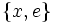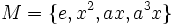# Retract not implies normal complements are isomorphic

## Statement

Suppose$G$ is a group and$H$ is a Retract (?) of$G$. In other words,$H$ has a Normal complement (?) in$G$: there exists a normal subgroup$N$ of$G$ such that$NH = G$ and$N \cap H$ is trivial.

Then, there may exist another normal complement$M$ to$H$ in$G$ such that$M$ is not isomorphic to$N$.

## Proof

### Example of the dihedral group

Further information: dihedral group:D8

Consider the following example:

•$G$ is the dihedral group of order eight:$\langle a,x \mid a^4 = x^2 = e, xax^{-1} = a^{-1} \rangle$.

•$H$ is the subgroup$\{ x, e \}$, i.e., a two-element cyclic subgroup.
• There are two normal complements to$H$ in$G$. The subgroup$N = \{ x, x^2, x^3, e \}$ is cyclic of order four and the subgroup$M = \{ e, x^2, ax, a^3x \}$ is a Klein-four group.